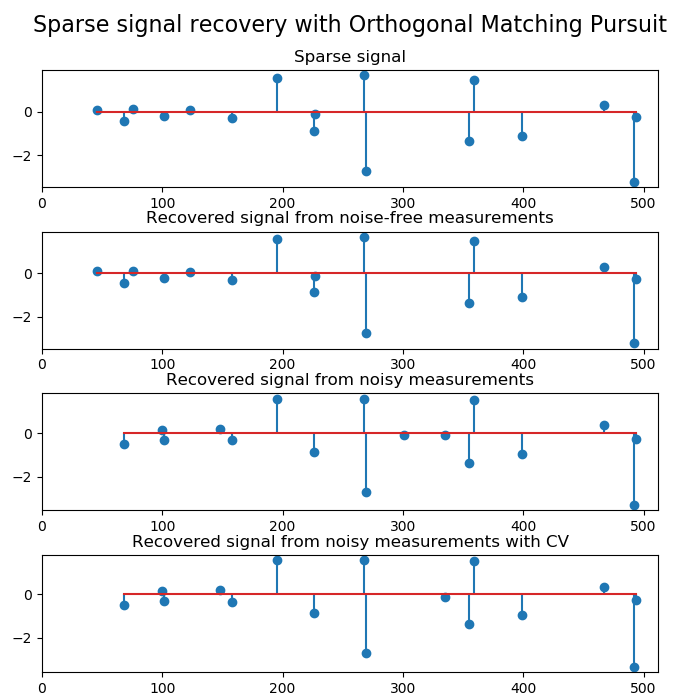# sklearn.linear_model.OrthogonalMatchingPursuit¶

class sklearn.linear_model.OrthogonalMatchingPursuit(*, n_nonzero_coefs=None, tol=None, fit_intercept=True, normalize=True, precompute='auto')

n_nonzero_coefs int, optional

tol float, optional

fit_intercept boolean, optional

normalize boolean, optional, default True
fit_intercept设置为False 时，将忽略此参数。如果为True，则在回归之前通过减去均值并除以l2-范数来对回归变量X进行归一化。如果你希望标准化，请先使用 sklearn.preprocessing.StandardScaler，然后调用fit 估算器并设置normalize=False
precompute {True, False, ‘auto’}, default ‘auto’

coef_ array, shape (n_features,) or (n_targets, n_features)

intercept_ float or array, shape (n_targets,)

n_iter_ int or array-like

>>> from sklearn.linear_model import OrthogonalMatchingPursuit>>> from sklearn.datasets import make_regression>>> X, y = make_regression(noise=4, random_state=0)>>> reg = OrthogonalMatchingPursuit().fit(X, y)>>> reg.score(X, y)0.9991...>>> reg.predict(X[:1,])array([-78.3854...])

fit(X, y[, copy_X]) 使用X，y作为训练数据拟合模型。
get_params([deep]) 获取此估计量的参数。
predict(X) 使用线性模型进行预测。
score(X, y[, sample_weight]) 返回预测的确定系数R ^ 2。
set_params(**params) 设置此估算器的参数。
__init__（standard ='aic'，*，fit_intercept = True，verbose = False，normalize = True，precompute ='auto'，max_iter = 500，eps = 2.220446049250313e-16，copy_X = True，positive = False ）

[源码]

fit(X, y, copy_X=None)

[源码]

X array-like of shape (n_samples, n_features) 训练数据。
y array-like of shape (n_samples,) 目标值。如有必要，将强制转换为X的数据类型
copy_X bool, default=None 如果设置了此参数，它将覆盖实例创建时选择的copy_X。如果True，X将被复制；否则X可能会被覆盖。

self object 返回估计器实例
get_params(deep=True)

[源码]

deep bool, default=True 如果为True，则将返回此估算器和所包含子对象的参数。

params mapping of string to any 参数名称映射到其值。
predict(X)

[源码]

X array_like or sparse matrix, shape (n_samples, n_features) 样本数据

C array, shape (n_samples,) 返回预测值。
score(X, y, sample_weight=None)

[源码]

X array-like of shape (n_samples, n_features) 测试样本。对于某些估计量，这可以是预先计算的内核矩阵或通用对象列表，形状为（n_samples，n_samples_fitted），其中n_samples_fitted是用于拟合估计器的样本数。
y array-like of shape (n_samples,) or (n_samples, n_outputs) X的真实值。
sample_weight array-like of shape (n_samples,), default=None 样本权重。

score float 预测值与真实值的R^2。

set_params(**params)

[源码]

**params dict 估计器参数。

self object 估计器实例。

## sklearn.linear_model.OrthogonalMatchingPursuit使用示例¶